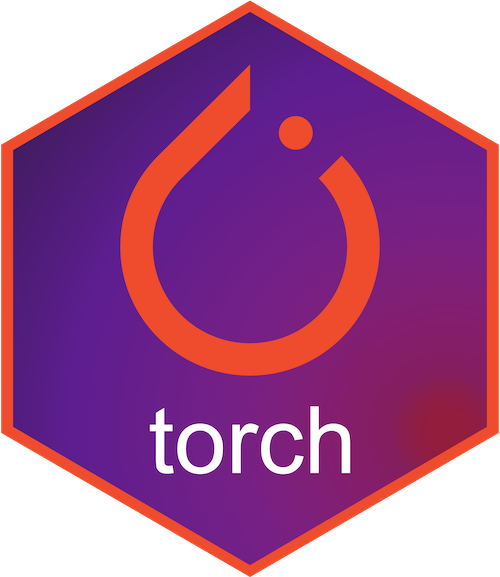# torch## Installation

torch can be installed from CRAN with:

``install.packages("torch")``

You can also install the development version with:

``remotes::install_github("mlverse/torch")``

## Examples

You can create torch tensors from R objects with the `torch_tensor` function and convert them back to R objects with `as_array`.

``````library(torch)
x <- array(runif(8), dim = c(2, 2, 2))
y <- torch_tensor(x, dtype = torch_float64())
y
#> torch_tensor
#> (1,.,.) =
#>   0.6192  0.5800
#>   0.2488  0.3681
#>
#> (2,.,.) =
#>   0.0042  0.9206
#>   0.4388  0.5664
#> [ CPUDoubleType{2,2,2} ]
identical(x, as_array(y))
#>  TRUE``````

In the following snippet we let torch, using the autograd feature, calculate the derivatives:

``````x <- torch_tensor(1, requires_grad = TRUE)
w <- torch_tensor(2, requires_grad = TRUE)
b <- torch_tensor(3, requires_grad = TRUE)
y <- w * x + b
y\$backward()
#> torch_tensor
#>  2
#> [ CPUFloatType{1} ]
No matter your current skills it’s possible to contribute to `torch` development. See the contributing guide for more information.